Next: About this document ... Up: lab_template Previous: lab_template

Subsections

# Taylor Polynomials

## Purpose

The purpose of this lab is to use Maple to introduce you to Taylor polynomial approximations to functions, including some applications.

## Getting Started

To assist you, there is a worksheet associated with this lab that contains examples and even solutions to some of the exercises. You can copy that worksheet to your home directory with the following command, which must be run in a terminal window, not in Maple.

cp /math/calclab/MA1023/Taylor_start.mws ~


You can copy the worksheet now, but you should read through the lab before you load it into Maple. Once you have read to the exercises, start up Maple, load the worksheet Taylor_start.mws, and go through it carefully. Then you can start working on the exercises.

## Background

The idea of the Taylor polynomial approximation of order
at, written
, to a smooth function
is to require thatandhave the same value at. Furthermore, their derivatives atmust match up to order. For example the Taylor polynomial of order three foratwould have to satisfy the conditions
You should check for yourself that the cubic polynomial satisfying these four conditions is
The general form of the Taylor polynomial approximation of ordertois given by the following

Theorem 1   Suppose thatis a smooth function in some open interval containing. Then theth degree Taylor polynomial of the functionat the pointis given byWe will be seeing this formula a lot, so it would be good for you to memorize it now! The notation
is used in the definition to stand for the value of the-th derivative of
at. That is,,
, and so on. By convention,. Note that
is fixed and so the derivativesare just numbers. That is, a Taylor polynomial has the formwhich you should recognize as a power series that has been truncated.

## Accuracy and Tolerance

To measure how well a Taylor Polynomial approximates the function over a specified interval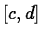, we define the toleranceofto be the maximum of the absolute error
over the interval. The Getting started worksheet has examples of how to compute and plot the absolute error.

## Exercises

1. For the following functions and base points, determine what minimum order is required so that the Taylor polynomial approximates the function to within a tolerance of
over the given interval.
1., base point, interval
.
2., base point, interval
.
3., base point, interval
.
4., base point, interval.

2. For the function,
, use the TayPlot command to plot the function and a Taylor polynomial approximation of order 5 with base pointon the same graph over the interval
. If you increase the order of the Taylor polynomial, can you get a good approximation at
? Can you make a good guess at the radius of convergence of the Taylor series for?

3. Consider the function. Plot the graph of this function along with its Taylor polynomial approximation of various orders with base pointover the interval. Limit the
range of your plot to. Can you make a good guess at the interval of convergence of the Taylor series?Next: About this document ... Up: lab_template Previous: lab_template
Dina Solitro
2004-11-22